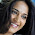Skip to main content

### Installing python modules without pip

Ever wanted to install python modules, but you were unable to do it using pip install command, or simply wanted to do it using python, if so then this is the post you want to read.
pip module is now by default installed when you install the latest python, if you want to install multiple python modules you can either enter pip commands in command prompt or terminal or make a batch file,
In this post, we will see how to install all the modules that you want by executing a python program.

Lets look at the code,

In the above code you can simply put the modules you want to install in the list and execute the code.

You might also be interested in

Data Encryption using Caesar Cipher
LCM of 2 numbers
Anagram Strings
Double Linked List
Finding Middle node in a Linked List
Infix to Prefix Conversion

### Comments

1.I like your blog, I read this blog please update more content on python, further check it once at python online training

### Infix to Prefix conversion using Stack

This post is about conversion of Infix expression to Prefix conversion. For this conversion we take help of stack data structure, we need to push and pop the operators in and out of the stack.

Infix expressions are the expressions that we normally use,eg. 5+6-7; a+b*c etc. Prefix expressions are the expressions in which the 2 operands are preceded by the operator eg. -+567 , +a*bc etc.

This method is very similar to the method that we used to convert Infix to Postfix but the only difference is that here we need to reverse the input string before conversion and then reverse the final output string before displaying it.

NOTE: This changes one thing that is instead of encountering the opening bracket we now first encounter the closing bracket and we make changes accordingly in our code.

So, to convert an infix expression to a prefix expression we follow the below steps
(we have 2 string, 1st is the input infix expression string 2nd is the output string which is empty initially)

We first revers…

### Hashing with Quadratic Probing

Hashing is a technique used for storing , searching and removing elements in almost constant time. Hashing is done with help of a hash function that generates index for a given input, then this index can be used to search the elements, store an element, or remove that element from that index.

A hash function is a function that is used to map the data elements to their position in the data structure used. For example if we use an array to store the integer elements then the hash function will generate position for each element so that searching, storing and removing operation on the array can be done in constant time that is independent of the number of elements in the array. For better look at the example below.

now we face a problem if for 2 numbers same position is generated example consider elements 1 and 14

1 % 13 = 1

14 % 13 = 1

so when we get 1 we store it at the first position, but when we get 14 we see that the position 1 is already taken, this is a case of collision.

Inorder…